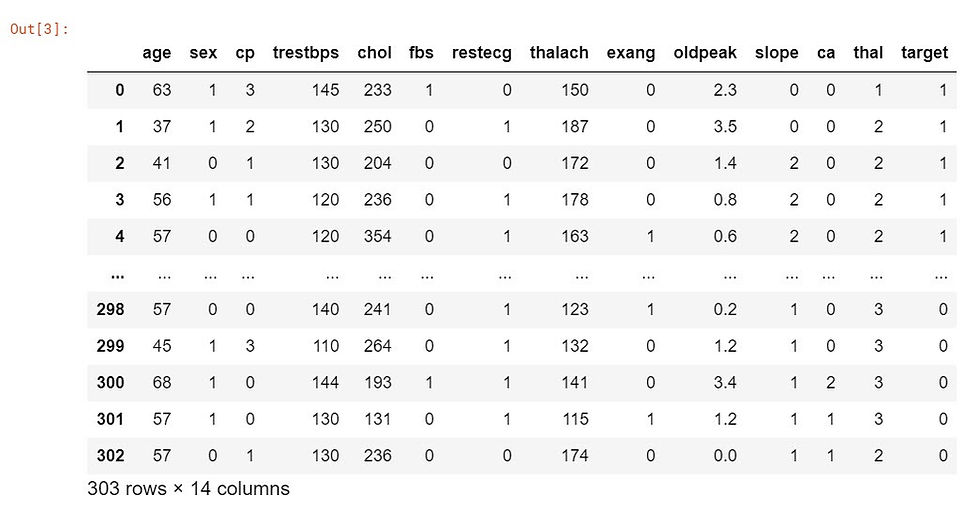top of pageSearch

# Example for XGBoost

XG Boost is very powerful Machine learning algorithm which can have higher rates of accuracy when specified by its wide range of parameters in supervised machine learning. XGBoost stands for eXtreme Gradient Boosting. XG Boost works on parallel tree boosting which predicts the target by combining results of multiple weak model. The XGBoost library implements the gradient boosting decision tree algorithm. Let us explore more using an example.

Here we are using heart disease uci data set from kaggle. Also we are trying to predict the likelihood of getting heart disease and which feature is more important for that.

Here is the link for data. Data

First let us import pandas to read the data using the following line of code

`import pandas as pd`

Now let us read the data to a dataframe

``` data = pd.read_csv('../input/heart-disease-uci/heart.csv')
data```

This will read the data into dataframe called data and gives the following output.Now let us see the info of the data to explore more about data using the following code

`data.info()`

This will give the following information about the data. We can see data types, columns, null values etc.

```<class 'pandas.core.frame.DataFrame'>
RangeIndex: 303 entries, 0 to 302
Data columns (total 14 columns):
#   Column    Non-Null Count  Dtype
---  ------    --------------  -----
0   age       303 non-null    int64
1   sex       303 non-null    int64
2   cp        303 non-null    int64
3   trestbps  303 non-null    int64
4   chol      303 non-null    int64
5   fbs       303 non-null    int64
6   restecg   303 non-null    int64
7   thalach   303 non-null    int64
8   exang     303 non-null    int64
9   oldpeak   303 non-null    float64
10  slope     303 non-null    int64
11  ca        303 non-null    int64
12  thal      303 non-null    int64
13  target    303 non-null    int64
dtypes: float64(1), int64(13)
memory usage: 33.3 KB```

Let us see another form of information through following code

`data.describe()`

This will give the description of the dataframe in the following way.Description gave count, mean, std, min, 25%, 50%, 75% and max of all columns. This further helps us to understand more about data. Now let us import the numpy, xgboost and sklearn.metrics libraries. We need mean squared error for regression.

``` import xgboost as xgb
from sklearn.metrics import mean_squared_error
import numpy as np ```

Next we need to assign X and Y values. Here we are also seperating the target variable and the rest of the variables using .iloc to subset the data..

`X, Y = data.iloc[:,:-1],data.iloc[:,-1]`

Now we need to convert the dataset into an optimized data structure called Dmatrix that XGBoost supports and gives it acclaimed performance and efficiency gains.

`data_dmatrix = xgb.DMatrix(data=X,label=Y)`

Now, we will create the train and test set for cross-validation of the results using the train_test_split function from sklearn's model_selection module with test_size size equal to 20% of the data. Also, to maintain reproducibility of the results, a random_state is also assigned .

``` from sklearn.model_selection import train_test_split
X_train, X_test, Y_train, Y_test = train_test_split(X, Y,
test_size=0.2, random_state=123)```

The next step is to instantiate an XGBoost regressor object by calling the XGBRegressor() class from the XGBoost library with the hyper-parameters passed as arguments.

`xg_reg = xgb.XGBRegressor(objective ='reg:linear', colsample_bytree = 0.3, learning_rate = 0.1,max_depth = 5, alpha = 10, n_estimators = 10)`

Now let us fit the regressor to the training set using the .fit() method.

` xg_reg.fit(X_train,Y_train)`

the output after fitting shows many hyperparameters as follows.

```XGBRegressor(alpha=10, base_score=0.5,                           booster='gbtree',colsample_bylevel=1,colsample_bynode=1, colsample_bytree=0.3, gamma=0, gpu_id=-1,importance_type='gain', interaction_constraints='',learning_rate=0.1, max_delta_step=0, max_depth=5,min_child_weight=1, missing=nan, monotone_constraints='()',
n_estimators=10, n_jobs=0, num_parallel_tree=1,objective='reg:linear', random_state=0, reg_alpha=10, reg_lambda=1,scale_pos_weight=1, subsample=1, tree_method='exact',validate_parameters=1, verbosity=None)```

Let us predict the above model using the .predict() method.

```preds = xg_reg.predict(X_test)
preds```

The out put is as follows

```array([0.62361383, 0.436409  , 0.4638252 , 0.4234442 , 0.5576368 ,
0.36139676, 0.6453048 , 0.48385164, 0.61654013, 0.53615165,
0.5271714 , 0.5342451 , 0.36847046, 0.36139676, 0.5126163 ,
0.42248273, 0.47246233, 0.52830946, 0.4810629 , 0.5204763 ,
0.590331  , 0.47579026, 0.6621046 , 0.39579174, 0.49821952,
0.53383183, 0.4865605 , 0.5409056 , 0.43799824, 0.53313845,
0.4234442 , 0.5955741 , 0.5409056 , 0.45628968, 0.5528887 ,
0.60049963, 0.61878484, 0.6078624 , 0.62996906, 0.59981364,
0.64041364, 0.55104494, 0.5254018 , 0.62684685, 0.55517054,
0.48204178, 0.36139676, 0.44480985, 0.60275817, 0.6277096 ,
0.44678292, 0.42796794, 0.6621046 , 0.5477844 , 0.49884164,
0.62160766, 0.55104494, 0.59651303, 0.5327918 , 0.55396104,
0.5179344 ], dtype=float32)```

Let us calculate the rmse by using the mean_sqaured_error function from sklearn's metrics module.The output will be as follows.

``` rmse = np.sqrt(mean_squared_error(Y_test, preds))
print("RMSE: %f" % (rmse))```
`RMSE: 0.449886`

We can see that RMSE prediction for heart disease came out to be 0.45.So our model can predict with this error. To analyse which feature is more important factor we need to classify and XGBclassifier() is used for that. Let us import the required libraries for this task.

``` from numpy import loadtxt
from xgboost import XGBClassifier
from xgboost import plot_importance
from matplotlib import pyplot```

Let us call the classifier using the following line

`model = XGBClassifier()`

Here we are trying to fit the model

`model.fit(X, Y)`

The above gives the following output with hyperparameters.

``` XGBClassifier(base_score=0.5, booster='gbtree', colsample_bylevel=1,
colsample_bynode=1, colsample_bytree=1,gamma=0,gpu_id=-1,
importance_type='gain', interaction_constraints='',
learning_rate=0.300000012, max_delta_step=0, max_depth=6,
min_child_weight=1,missing=nan,monotone_constraints='()',
n_estimators=100,n_jobs=0,num_parallel_tree=1,random_state=0,
reg_alpha=0, reg_lambda=1,scale_pos_weight=1,subsample=1,
tree_method='exact',validate_parameters=1,verbosity=None)

```

Here we are trying to plot the importance model using this code

``` plot_importance(model)
pyplot.show()```

The output shows the order of importanceConclusion

We have built XGBoost model for predicting the likelihood of getting heart disease and the model has 0.45 rmse. We have also found that the most important factor in finding out the heart disease is cholesterol.